# PAGE 29 Rock 1 Rock 2 Rock 3

• Slides: 12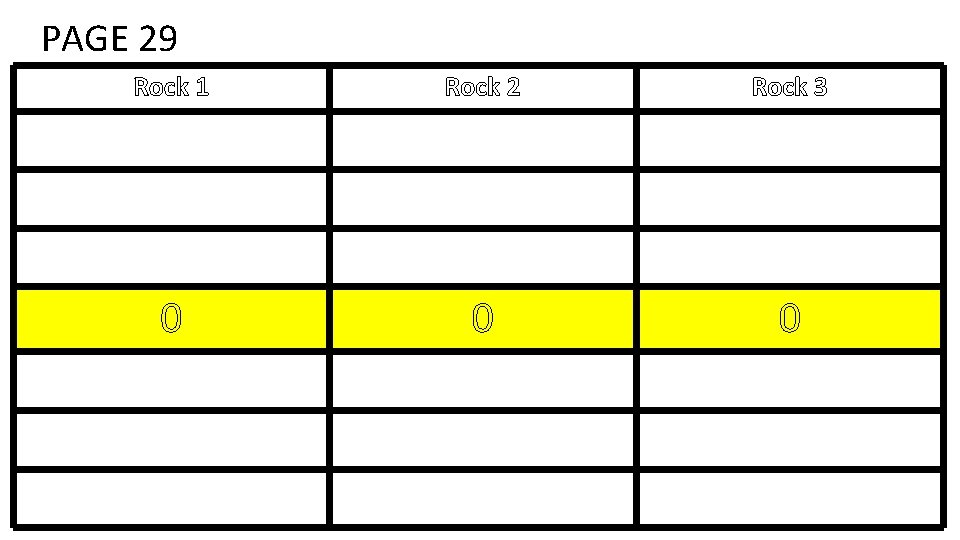PAGE 29 Rock 1 Rock 2 Rock 3 0 0 0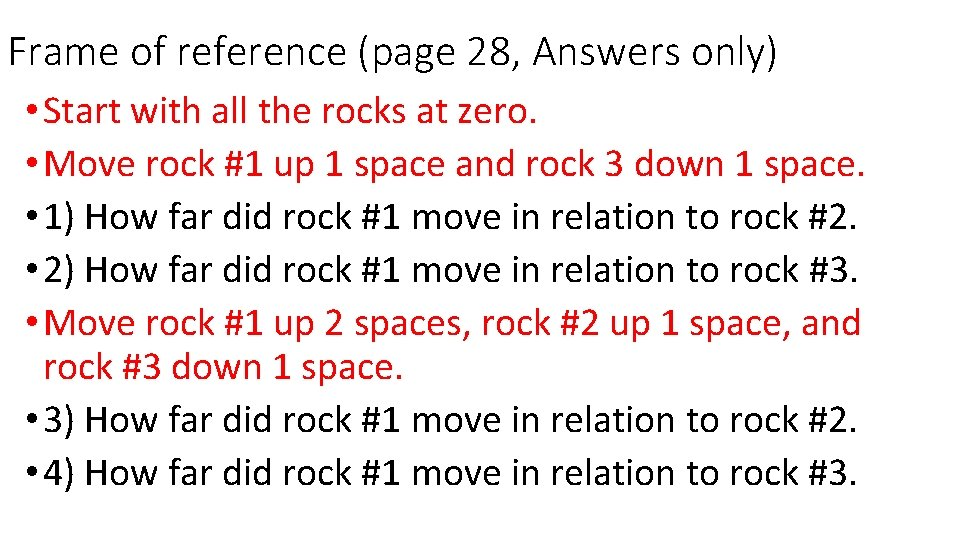Frame of reference (page 28, Answers only) • Start with all the rocks at zero. • Move rock #1 up 1 space and rock 3 down 1 space. • 1) How far did rock #1 move in relation to rock #2. • 2) How far did rock #1 move in relation to rock #3. • Move rock #1 up 2 spaces, rock #2 up 1 space, and rock #3 down 1 space. • 3) How far did rock #1 move in relation to rock #2. • 4) How far did rock #1 move in relation to rock #3.Page 29 of INB (under frame of reference) • Step 1: place the ramp with 2 books under the starting point. • Step 2: role the marble down the ramp and measure the time to reach the finish line. • Step 3: repeat steps 1 and 2 raising the ramp by one book each time. Total Distance 2 Books 3 Books 4 books Time SPEED (d/t)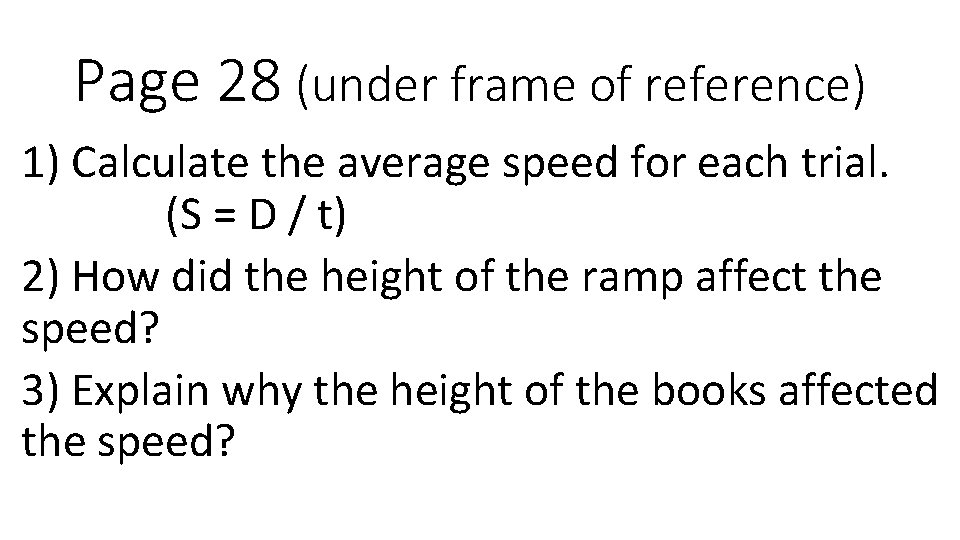Page 28 (under frame of reference) 1) Calculate the average speed for each trial. (S = D / t) 2) How did the height of the ramp affect the speed? 3) Explain why the height of the books affected the speed?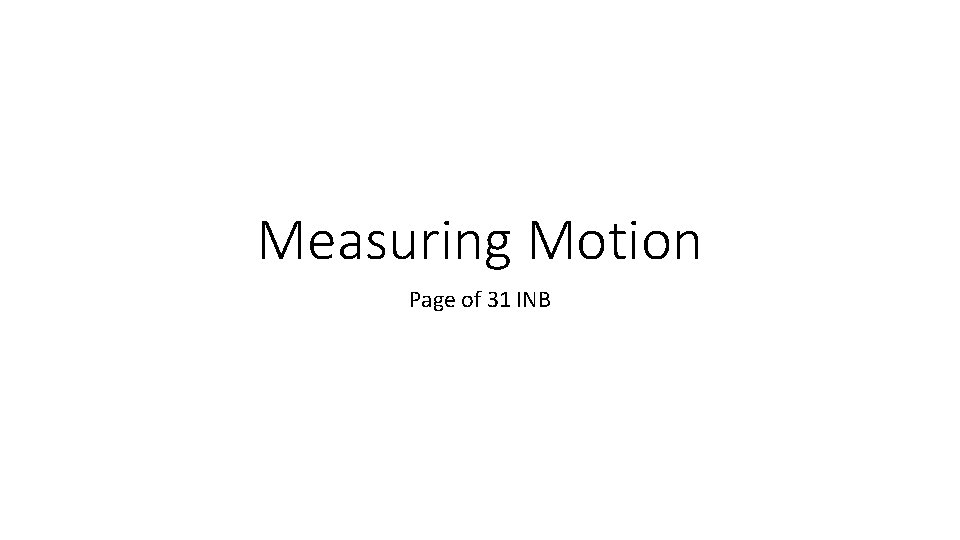Measuring Motion Page of 31 INB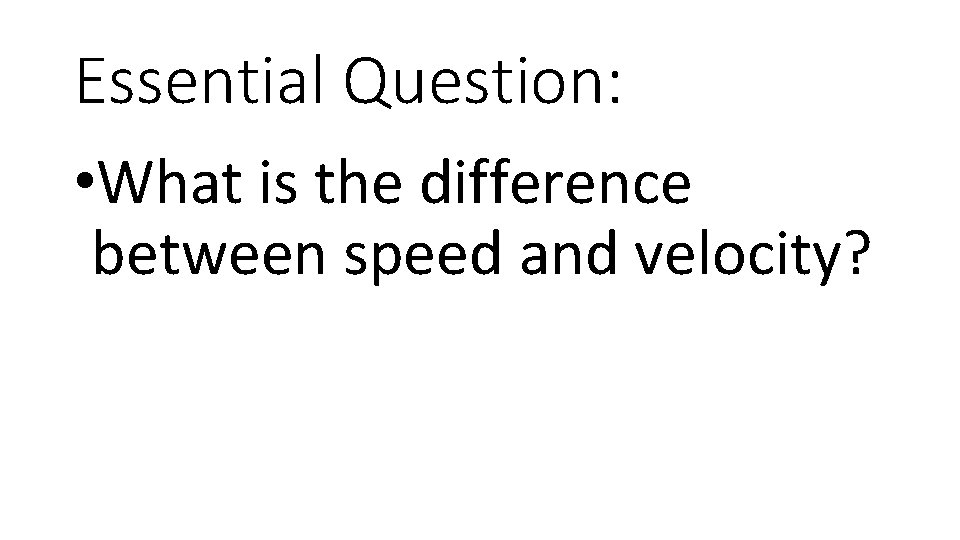Essential Question: • What is the difference between speed and velocity?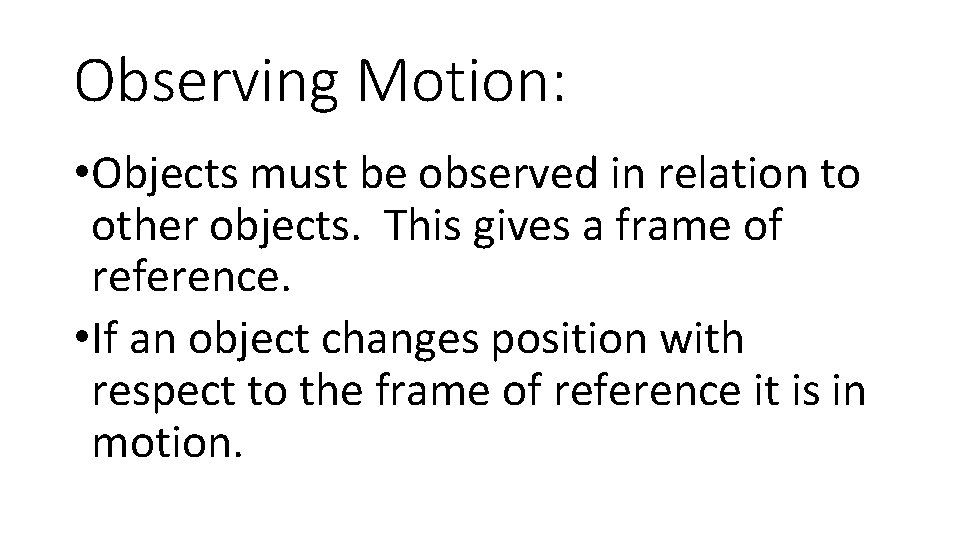Observing Motion: • Objects must be observed in relation to other objects. This gives a frame of reference. • If an object changes position with respect to the frame of reference it is in motion.Distance vs Displacement • Distance is the measure of the actual path taken. • Displacement is the measure from start to finish. • Displacement must include a direction.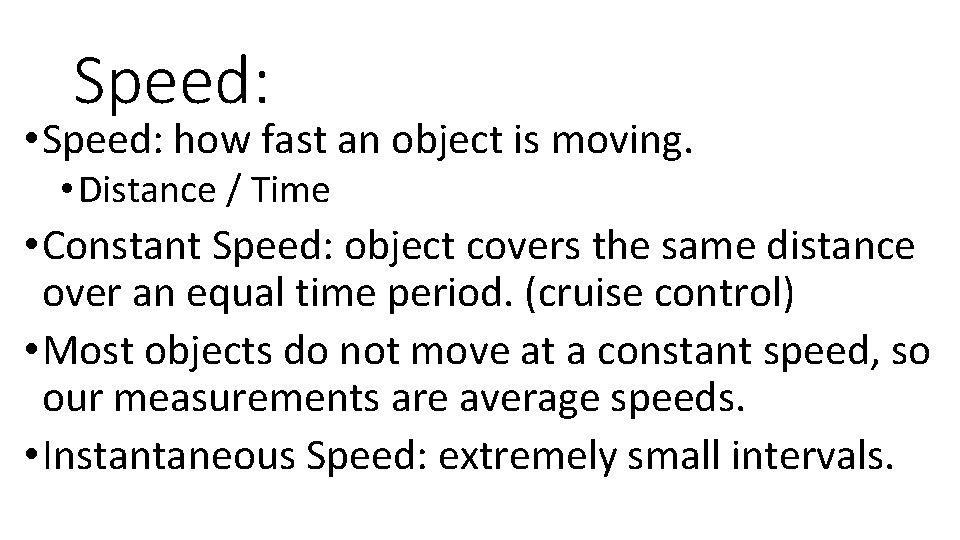Speed: • Speed: how fast an object is moving. • Distance / Time • Constant Speed: object covers the same distance over an equal time period. (cruise control) • Most objects do not move at a constant speed, so our measurements are average speeds. • Instantaneous Speed: extremely small intervals.Speed vs Velocity • Velocity is speed with a given direction. • Describe relative to a reference point. • Can be combined. (kid on a school bus).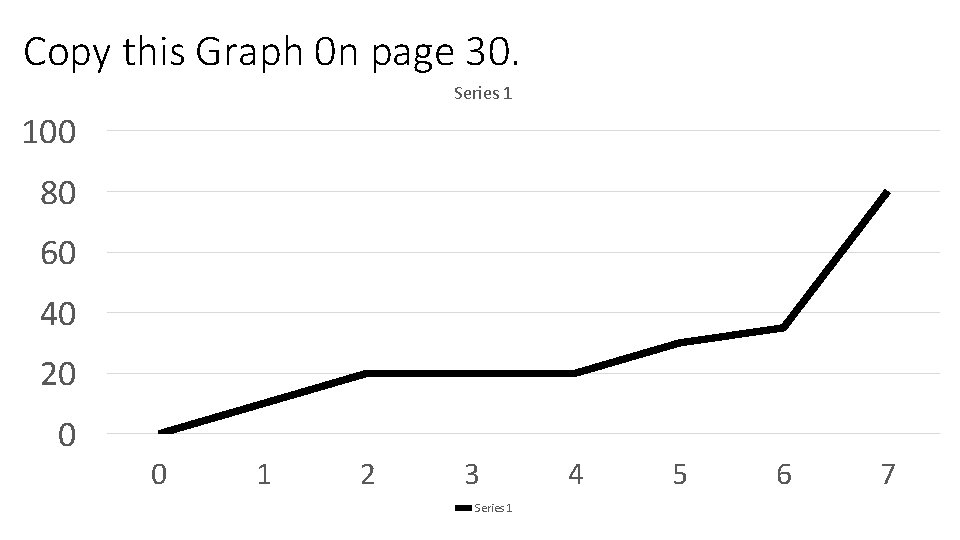Copy this Graph 0 n page 30. Series 1 100 80 60 40 20 0 0 1 2 3 Series 1 4 5 6 7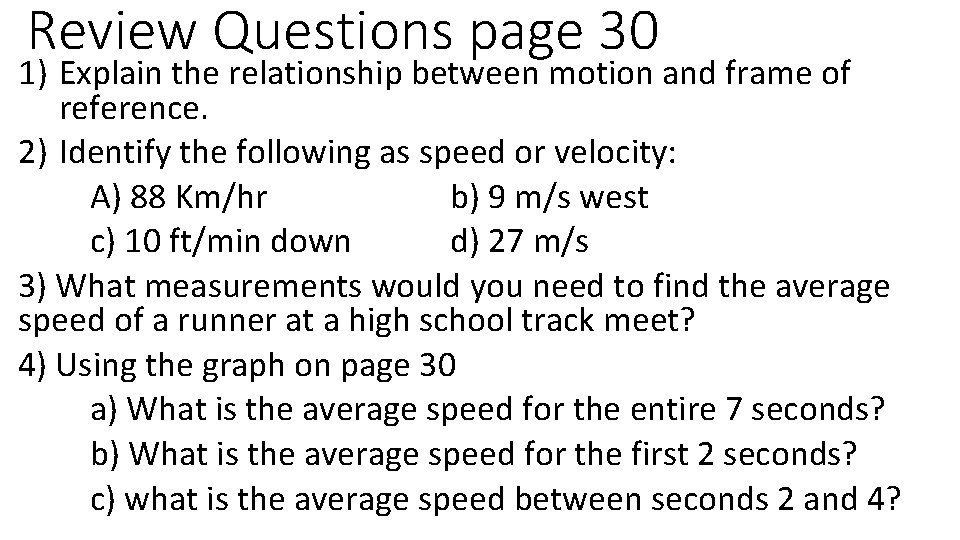Review Questions page 30 1) Explain the relationship between motion and frame of reference. 2) Identify the following as speed or velocity: A) 88 Km/hr b) 9 m/s west c) 10 ft/min down d) 27 m/s 3) What measurements would you need to find the average speed of a runner at a high school track meet? 4) Using the graph on page 30 a) What is the average speed for the entire 7 seconds? b) What is the average speed for the first 2 seconds? c) what is the average speed between seconds 2 and 4?